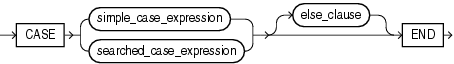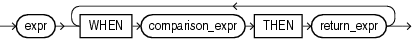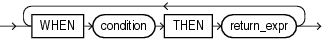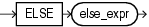# CASE Expressions

`CASE` expressions let you use `IF` ... `THEN` ... `ELSE` logic in SQL statements without having to invoke procedures. The syntax is:Description of the illustration case_expression.gif

simple_case_expression::=Description of the illustration simple_case_expression.gif

searched_case_expression::=Description of the illustration searched_case_expression.gif

else_clause::=Description of the illustration else_clause.gif

In a simple `CASE` expression, Oracle Database searches for the first `WHEN` ... `THEN` pair for which `expr` is equal to `comparison_expr` and returns `return_expr`. If none of the `WHEN` ... `THEN` pairs meet this condition, and an `ELSE` clause exists, then Oracle returns `else_expr`. Otherwise, Oracle returns null. You cannot specify the literal `NULL` for every `return_expr` and the `else_expr`.

In a searched `CASE` expression, Oracle searches from left to right until it finds an occurrence of `condition` that is true, and then returns `return_expr`. If no `condition` is found to be true, and an `ELSE` clause exists, then Oracle returns `else_expr`. Otherwise, Oracle returns null.

Oracle Database uses short-circuit evaluation. For a simple `CASE` expression, the database evaluates each `comparison_expr` value only before comparing it to `expr`, rather than evaluating all `comparison_expr` values before comparing any of them with `expr`. Consequently, Oracle never evaluates a `comparison_expr` if a previous `comparison_expr` is equal to `expr`. For a searched `CASE` expression, the database evaluates each `condition` to determine whether it is true, and never evaluates a `condition` if the previous `condition` was true.

For a simple `CASE` expression, the `expr` and all `comparison_expr` values must either have the same datatype (`CHAR`, `VARCHAR2`, `NCHAR`, or `NVARCHAR2`, `NUMBER`, `BINARY_FLOAT`, or `BINARY_DOUBLE`) or must all have a numeric datatype. If all expressions have a numeric datatype, then Oracle determines the argument with the highest numeric precedence, implicitly converts the remaining arguments to that datatype, and returns that datatype.

For both simple and searched `CASE` expressions, all of the `return_expr`s must either have the same datatype (`CHAR`, `VARCHAR2`, `NCHAR`, or `NVARCHAR2`, `NUMBER`, `BINARY_FLOAT`, or `BINARY_DOUBLE`) or must all have a numeric datatype. If all return expressions have a numeric datatype, then Oracle determines the argument with the highest numeric precedence, implicitly converts the remaining arguments to that datatype, and returns that datatype.

The maximum number of arguments in a `CASE` expression is 255. All expressions count toward this limit, including the initial expression of a simple `CASE` expression and the optional `ELSE` expression. Each `WHEN` ... `THEN` pair counts as two arguments. To avoid exceeding this limit, you can nest `CASE` expressions so that the `return_expr` itself is a `CASE` expression.

Simple CASE Example For each customer in the sample `oe.customers` table, the following statement lists the credit limit as "Low" if it equals \$100, "High" if it equals \$5000, and "Medium" if it equals anything else.

```SELECT cust_last_name,
CASE credit_limit WHEN 100 THEN 'Low'
WHEN 5000 THEN 'High'
ELSE 'Medium' END AS credit
FROM customers
ORDER BY cust_last_name, credit;

CUST_LAST_NAME       CREDIT
-------------------- ------
Alexander            Medium
Alexander            Medium
Altman               High
Altman               Medium
. . .
```

Searched CASE Example The following statement finds the average salary of the employees in the sample table `oe.employees`, using \$2000 as the lowest salary possible:

```SELECT AVG(CASE WHEN e.salary > 2000 THEN e.salary
ELSE 2000 END) "Average Salary" FROM employees e;

Average Salary
--------------
6461.68224
```